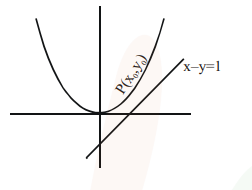# The shortest distance between the line

Question:

The shortest distance between the line $x-y=1$ and the curve $x^{2}=2 y$ is :

1. $\frac{1}{2}$

2. $\frac{1}{2 \sqrt{2}}$

3. $\frac{1}{\sqrt{2}}$

4. 0

Correct Option: , 2

Solution:Shortest distance between curves is always along common normal.

$\left.\frac{d y}{d x}\right|_{P}=$ slope of line $=1$

$\Rightarrow x_{0}=1$                           $\therefore \mathrm{y}_{0}=\frac{1}{2}$

$\Rightarrow \mathrm{P}\left(1, \frac{1}{2}\right)$

$\therefore$ Shortest distance $=\left|\frac{1-\frac{1}{2}-1}{\sqrt{1^{2}+1^{2}}}\right|=\frac{1}{2 \sqrt{2}}$